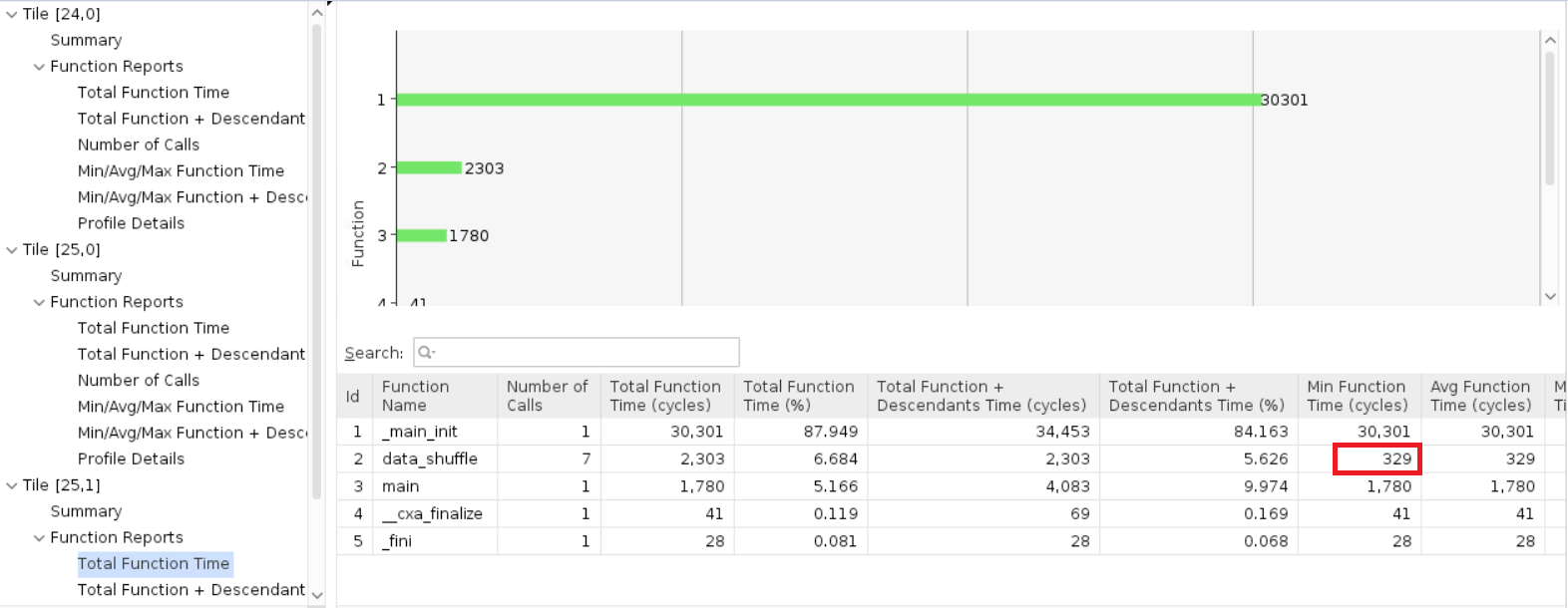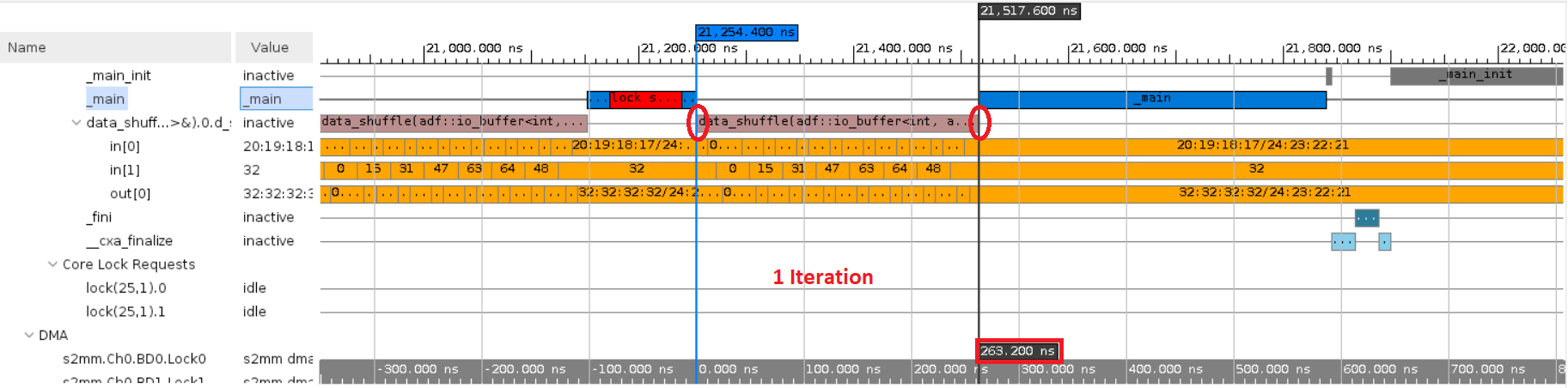# Calculating the Kernel Latency - 2023.2 English

## Vitis Tutorials: AI Engine

Document ID
XD100
Release Date
2023-11-29
Version
2023.2 English

From the Profile information in the Vitis Analyzer, analyze the function time of the kernels as explained in Section 9 in the AIE simulation.

For example, compare the function time of the `data_shuffle` kernel with the standalone AIE simulation result, and calculate the kernel latency.From the trace information, you can calculate the kernel latency as follows:

1. Click the `Trace` in the AI Engine simulation run summary, and navigate to the any function to calculate the latency. For example, consider the `data_shuffle` function.

2. You can notice the function `data_shuffle` ran for seven iterations. Zoom into the period of one iteration (between two `main()` function calls as follows), add a marker, and drag it to the end of the kernel function as follows:Notice the difference of `263.2 ns` as highlighted above. This is the time the kernel took to complete one iteration.

If you click the AI Engine Simulation Summary, you can notice the AI Engine Frequency as 1250 MHz, i.e., 0.8 ns, i.e., one cycle = 0.8 ns. Now, the data_shuffle function took 263.2 ns for one iteration, i.e., 263.2 / 0.8 ~= 329 cycles.

Compare this with the latency you got during the `aiesimulation` where the AI Engine is a standalone module; see Section-9 in AIE Simulation.